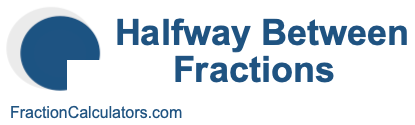Halfway between 1/10 and 3/6Here we will show you how to calculate the fraction exactly halfway between 1/10 and 3/6. In other words, what fraction is exactly in the middle of 1/10 and 3/6? Calculating the fraction exactly halfway between 1/10 and 3/6 is a three-step process. Here are the steps:

Step 1) Calculate the difference
The first step is to find the absolute difference between 1/10 and 3/6. We do that by subtracting 3/6 from 1/10, like this:

1/10 - 3/6 = |2/5|

Step 2) Divide the difference by two
Next, we divide the absolute difference by two. This gives us half of the absolute difference. To do this, we divide the answer in step 1 by 2, like this:

2/5 ÷ 2 = 1/5

Step 3) Add half the difference
In the final step, we add half of the absolute difference to the lowest fraction. The lowest fraction of 1/10 and 3/6 is 1/10. Therefore, we add 1/5 to 1/10, like this:

1/10 + 1/5 = 3/10

There you have it, folks. Per our calculations above, the fraction exactly halfway between 1/10 and 3/6 is as follows:

3/10

Halfway Between Fractions Calculator
You can use this tool to calculate the halfway point between another set of fractions.

Halfway between
/
and
/

What fraction is halfway between 1/10 and 3/7?
Here is a similar fraction math problem that we have explained and solved for you!